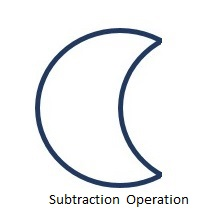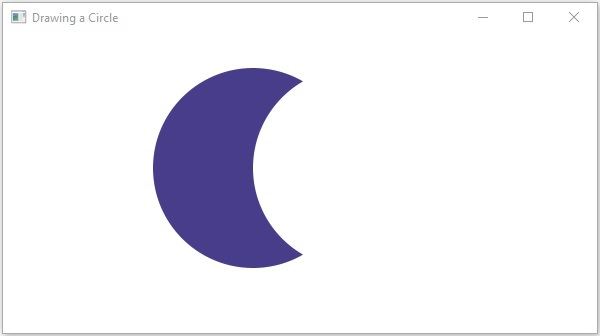# 2D Shapes(Objects) Subtraction Operation

This operation takes two or more shapes as an input. Then, it returns the area of the first shape excluding the area overlapped by the second one as shown below.You can perform the Subtraction Operation on the shapes using the method named subtract(). Since this is a static method, you should call it using the class name (Shape or its subclasses) as shown below.

```Shape shape = Shape.subtract(circle1, circle2);
```

Following is an example of the Subtraction Operation. In here, we are drawing two circles and performing a subtraction operation on them.

Save this code in a file with name SubtractionExample.java.

## Example

```import javafx.application.Application;
import javafx.scene.Group;
import javafx.scene.Scene;
import javafx.scene.paint.Color;
import javafx.stage.Stage;
import javafx.scene.shape.Circle;
import javafx.scene.shape.Shape;

public class SubtractionExample extends Application {
@Override
public void start(Stage stage) {
//Drawing Circle1
Circle circle1 = new Circle();

//Setting the position of the circle
circle1.setCenterX(250.0f);
circle1.setCenterY(135.0f);

//Setting the radius of the circle

//Setting the color of the circle
circle1.setFill(Color.DARKSLATEBLUE);

//Drawing Circle2
Circle circle2 = new Circle();

//Setting the position of the circle
circle2.setCenterX(350.0f);
circle2.setCenterY(135.0f);

//Setting the radius of the circle

//Setting the color of the circle
circle2.setFill(Color.BLUE);

//Performing subtraction operation on the circle
Shape shape = Shape.subtract(circle1, circle2);

//Setting the fill color to the result
shape.setFill(Color.DARKSLATEBLUE);

//Creating a Group object
Group root = new Group(shape);

//Creating a scene object
Scene scene = new Scene(root, 600, 300);

//Setting title to the Stage
stage.setTitle("Subtraction Example");

stage.setScene(scene);

//Displaying the contents of the stage
stage.show();
}
public static void main(String args[]){
launch(args);
}
}
```

Compile and execute the saved java file from the command prompt using the following commands.

```javac SubtractionExample.java
java SubtractionExample
```

On executing, the above program generates a JavaFX window displaying the following output −javafx_2d_shapes.htm